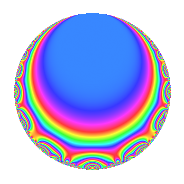Properties

 Label 2003.1.b.bLevel 2003 Weight 1 Character orbit 2003.b Self dual Yes Analytic conductor 1.000 Analytic rank 0 Dimension 3 Projective image $$D_{9}$$ CM disc. -2003 Inner twists 2

Related objects

Newspace parameters

 Level: $$N$$ = $$2003$$ Weight: $$k$$ = $$1$$ Character orbit: $$[\chi]$$ = 2003.b (of order $$2$$ and degree $$1$$)

Newform invariants

 Self dual: Yes Analytic conductor: $$0.999627220304$$ Analytic rank: $$0$$ Dimension: $$3$$ Coefficient field: $$\Q(\zeta_{18})^+$$ Coefficient ring: $$\Z[a_1, a_2, a_3]$$ Coefficient ring index: $$1$$ Projective image $$D_{9}$$ Projective field Galois closure of 9.1.16096216216081.1 Artin image size $$18$$ Artin image $D_9$ Artin field Galois closure of 9.1.16096216216081.1

$q$-expansion

Coefficients of the $$q$$-expansion are expressed in terms of a basis $$1,\beta_1,\beta_2$$ for the coefficient ring described below. We also show the integral $$q$$-expansion of the trace form.

 $$f(q)$$ $$=$$ $$q$$ $$-\beta_{1} q^{3}$$ $$+ q^{4}$$ $$+ ( 1 + \beta_{2} ) q^{9}$$ $$+O(q^{10})$$ $$q$$ $$-\beta_{1} q^{3}$$ $$+ q^{4}$$ $$+ ( 1 + \beta_{2} ) q^{9}$$ $$-\beta_{1} q^{12}$$ $$+ ( \beta_{1} - \beta_{2} ) q^{13}$$ $$+ q^{16}$$ $$- q^{19}$$ $$+ q^{25}$$ $$+ ( -1 - \beta_{1} ) q^{27}$$ $$+ ( 1 + \beta_{2} ) q^{36}$$ $$+ ( -1 + \beta_{1} - \beta_{2} ) q^{39}$$ $$+ ( \beta_{1} - \beta_{2} ) q^{47}$$ $$-\beta_{1} q^{48}$$ $$+ q^{49}$$ $$+ ( \beta_{1} - \beta_{2} ) q^{52}$$ $$- q^{53}$$ $$+ \beta_{1} q^{57}$$ $$+ \beta_{2} q^{59}$$ $$+ q^{64}$$ $$+ \beta_{2} q^{73}$$ $$-\beta_{1} q^{75}$$ $$- q^{76}$$ $$+ \beta_{2} q^{79}$$ $$+ ( 1 + \beta_{1} ) q^{81}$$ $$- q^{89}$$ $$+O(q^{100})$$ $$\operatorname{Tr}(f)(q)$$ $$=$$ $$3q$$ $$\mathstrut +\mathstrut 3q^{4}$$ $$\mathstrut +\mathstrut 3q^{9}$$ $$\mathstrut +\mathstrut O(q^{10})$$ $$3q$$ $$\mathstrut +\mathstrut 3q^{4}$$ $$\mathstrut +\mathstrut 3q^{9}$$ $$\mathstrut +\mathstrut 3q^{16}$$ $$\mathstrut -\mathstrut 3q^{19}$$ $$\mathstrut +\mathstrut 3q^{25}$$ $$\mathstrut -\mathstrut 3q^{27}$$ $$\mathstrut +\mathstrut 3q^{36}$$ $$\mathstrut -\mathstrut 3q^{39}$$ $$\mathstrut +\mathstrut 3q^{49}$$ $$\mathstrut -\mathstrut 3q^{53}$$ $$\mathstrut +\mathstrut 3q^{64}$$ $$\mathstrut -\mathstrut 3q^{76}$$ $$\mathstrut +\mathstrut 3q^{81}$$ $$\mathstrut -\mathstrut 3q^{89}$$ $$\mathstrut +\mathstrut O(q^{100})$$

Basis of coefficient ring in terms of $$\nu = \zeta_{18} + \zeta_{18}^{-1}$$:

 $$\beta_{0}$$ $$=$$ $$1$$ $$\beta_{1}$$ $$=$$ $$\nu$$ $$\beta_{2}$$ $$=$$ $$\nu^{2} - 2$$
 $$1$$ $$=$$ $$\beta_0$$ $$\nu$$ $$=$$ $$\beta_{1}$$ $$\nu^{2}$$ $$=$$ $$\beta_{2}\mathstrut +\mathstrut$$ $$2$$

Character Values

We give the values of $$\chi$$ on generators for $$\left(\mathbb{Z}/2003\mathbb{Z}\right)^\times$$.

 $$n$$ $$5$$ $$\chi(n)$$ $$-1$$

Embeddings

For each embedding $$\iota_m$$ of the coefficient field, the values $$\iota_m(a_n)$$ are shown below.

For more information on an embedded modular form you can click on its label.

Label $$\iota_m(\nu)$$ $$a_{2}$$ $$a_{3}$$ $$a_{4}$$ $$a_{5}$$ $$a_{6}$$ $$a_{7}$$ $$a_{8}$$ $$a_{9}$$ $$a_{10}$$
2002.1
 1.87939 −0.347296 −1.53209
0 −1.87939 1.00000 0 0 0 0 2.53209 0
2002.2 0 0.347296 1.00000 0 0 0 0 −0.879385 0
2002.3 0 1.53209 1.00000 0 0 0 0 1.34730 0
 $$n$$: e.g. 2-40 or 990-1000 Significant digits: Format: Complex embeddings Normalized embeddings Satake parameters Satake angles

Inner twists

Char. orbit Parity Mult. Self Twist Proved
1.a Even 1 trivial yes
2003.b Odd 1 CM by $$\Q(\sqrt{-2003})$$ yes

Hecke kernels

This newform can be constructed as the kernel of the linear operator $$T_{3}^{3}$$ $$\mathstrut -\mathstrut 3 T_{3}$$ $$\mathstrut +\mathstrut 1$$ acting on $$S_{1}^{\mathrm{new}}(2003, [\chi])$$.